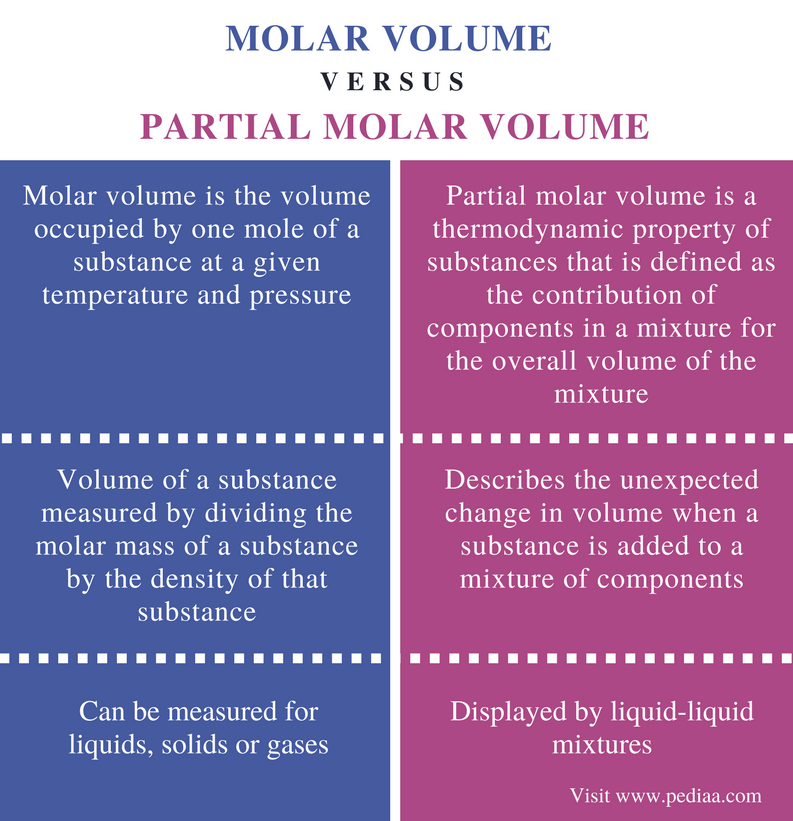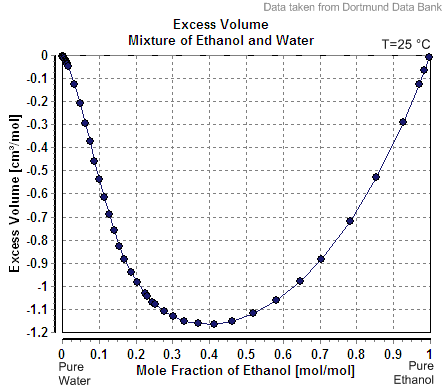# Difference Between Molar Volume and Partial Molar Volume

## Main Difference – Molar Volume vs Partial Molar Volume

Molar volume is the volume of one mole of a substance. The molar volume is denoted by the symbol Vm. At standard temperature and pressure, the molar volume of ideal gas is 22.4 L/mol. But for other substances, molar volume is highly dependent on the temperature and pressure in which the volume is measured. On the other hand, the partial molar volume is a complicated term. It can be defined as the contribution of a component of a mixture for the overall volume of the mixture. The main difference between molar volume and partial molar volume is that molar volume of a substance is the volume of one mole of that substance whereas partial molar volume is the change in volume of a mixture when a component is added to that mixture.

### Key Areas Covered

1. What is Molar Volume
– Definition, Molar Volume of Ideal Gases, Units of Measurement, Calculation
2. What is Partial Molar Volume
– Definition, Explanation with an Example
3. What is the Difference Between Molar Volume and Partial Molar Volume
– Comparison of Key Differences

Key Terms: Density, Ethanol, Ideal Gas, Molar Mass, Molar Volume, Partial Molar Volume, Temperature, Volume## What is Molar Volume

Molar volume is the volume occupied by one mole of a substance at a given temperature and pressure. The substance can be in gaseous form, liquid form or solid form. The chemical symbol for molar volume is Vm. The molar volume of an ideal gas at standard temperature and pressure is 22.4 L/mol.

### Molar Volume Calculation

For ideal behavior, PV  =  nRT

The standard values for temperature and pressure are 273 K and 1.00 atm respectively. Then the molar volume of ideal gas is,

(1.00 atm) x Vm    =    (1 mol) x (0.08206 L atm mol-1 K-1) x (273 K)

Vm    =    22.4 L/mol

The SI unit for the molar volume is cubic meters per mole (m3/mol). But in general, cubic decimeters per mole (dm3/mol) is used for gases and cubic centimeters per mole (cm3/mol) for liquids and solid.

Molar volume = Molar mass / Density

The molar volume of a substance can be simply calculated using the above relation measuring molar mass and the density of the substance. But if it is a mixture of several components, it can be simply the sum of the individual components. But some liquid mixtures violate this concept.Figure 01: Excess Volume Mixture of Ethanol and Water

For example, when water and ethanol are mixed, the calculated molar volume is not the sum of the values of molar volumes of each; it is a decreased value. This happens due to the formation of intermolecular forces between water molecules and ethanol molecules.

## What is Partial Molar Volume

Partial molar volume is a thermodynamic property of substances that is defined as the contribution of components in a mixture for the overall volume of the mixture. It is simply the change in volume when one mole of a component is added to a mixture. Let us consider an example to understand this concept.

• When a small amount of water is added to a large amount of water at 25oC, the volume of water is increased by 18.07 cm3/mol. But when water is added to ethanol, the volume of the mixture is increased only by 14 cm3/mol at the same temperature and pressure.
• This is because of the hydrogen bond formation between ethanol molecules and water molecules. Ethanol molecules have –OH groups that are capable of forming hydrogen bonds. When hydrogen bonds are formed, the molecules get closer, and the volume is decreased than expected.
• Hence, the partial molar volume of water in ethanol at 25oC is 14cm3/mol.Figure 1: A Curve Showing the Partial Molar Volume of a Mixture.

The above image shows the partial molar volume of a mixture containing two components: A and B. The dotted line in the graph shows the expected volume. But the actual volume has been decreased by ΔVmix.

## Difference Between Molar Volume and Partial Molar Volume

### Definition

Molar Volume: Molar volume is the volume occupied by one mole of a substance at a given temperature and pressure.

Partial Molar Volume: Partial molar volume is a thermodynamic property of substances that is defined as the contribution of components in a mixture for the overall volume of the mixture.

### Principle

Molar Volume: Molar volume is the volume of a substance measured by dividing the molar mass of a substance by the density of that substance.

Partial Molar Volume: Partial molar volume describes the unexpected change in volume when a substance is added to a mixture of components.

### Substances

Molar Volume: Molar volume can be measured for liquids, solids or gases.

Partial Molar Volume: Partial molar volume is displayed by liquid-liquid mixtures.

### Conclusion

Molar volume and partial molar volume are two related terms in thermodynamics. Both these terms describe the change in volume of a system per mole. The main difference between molar volume and partial molar volume is that molar volume of a substance is the volume of one mole of that substance whereas partial molar volume is the change in volume of a mixture when a component is added to that mixture.

##### Reference:

1. Helmenstine, Anne Marie. “Molar Volume Definition.” ThoughtCo, Jun. 13, 2014, Available here.
2. “Molar volume.” Wikipedia, Wikimedia Foundation, 26 Dec. 2017, Available here.
3. “Partial Molar Volume.” Partial Molar Volume – Everyscience.Com, Available here.

##### Image Courtesy:

1. “Excess Volume Mixture of Ethanol and Water” By Wilfried Cordes – de:Dortmunder Datenbank; en:Dortmund Data Bank (CC BY-SA 3.0) via Commons Wikimedia
2. “Partial molar volume EN” By Woudloper – Own work (CC BY-SA 3.0) via Commons Wikimedia